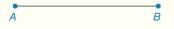Chapter 1.CT, Problem 19CT### Elementary Geometry for College St...

6th Edition
Daniel C. Alexander + 1 other
ISBN: 9781285195698

#### Solutions

Chapter
Section### Elementary Geometry for College St...

6th Edition
Daniel C. Alexander + 1 other
ISBN: 9781285195698
Textbook Problem
1 views

# Construct the perpendicular bisector of A B ¯ .To determine

To find:

The complete construction of the perpendicular bisector of AB¯.

Explanation

Given:

A line segment AB¯ is given.

Approach:

A line segment AB¯ is given.

The steps are given below for the complete construction.

a) Use A and B as the centers for the compass and take the radius greater than the length of 12BA.

b) These arcs intersect above the AB¯ and marked the intersection point as D.

c) Use A and B as the centers for the compass and take the radius greater than the length of 12BA.

d) These arcs intersect below the AB¯ and marked the intersection point as C

### Still sussing out bartleby?

Check out a sample textbook solution.

See a sample solution

#### The Solution to Your Study Problems

Bartleby provides explanations to thousands of textbook problems written by our experts, many with advanced degrees!

Get Started

#### In problems 15-26, evaluate each expression. 22.

Mathematical Applications for the Management, Life, and Social Sciences

#### In Exercises 1124, find the indicated limits, if they exist. 16. limx2x22x3x2+5+6

Applied Calculus for the Managerial, Life, and Social Sciences: A Brief Approach

#### Convert the expressions in Exercises 8596 radical form. 45x3/2

Finite Mathematics and Applied Calculus (MindTap Course List)